## Recommended Posts

Newbie alert.  Having trouble figuring out how to select certain points procedurally on the roof system.  I would like to group specific points then ray them onto bounding region to get the attached desired look.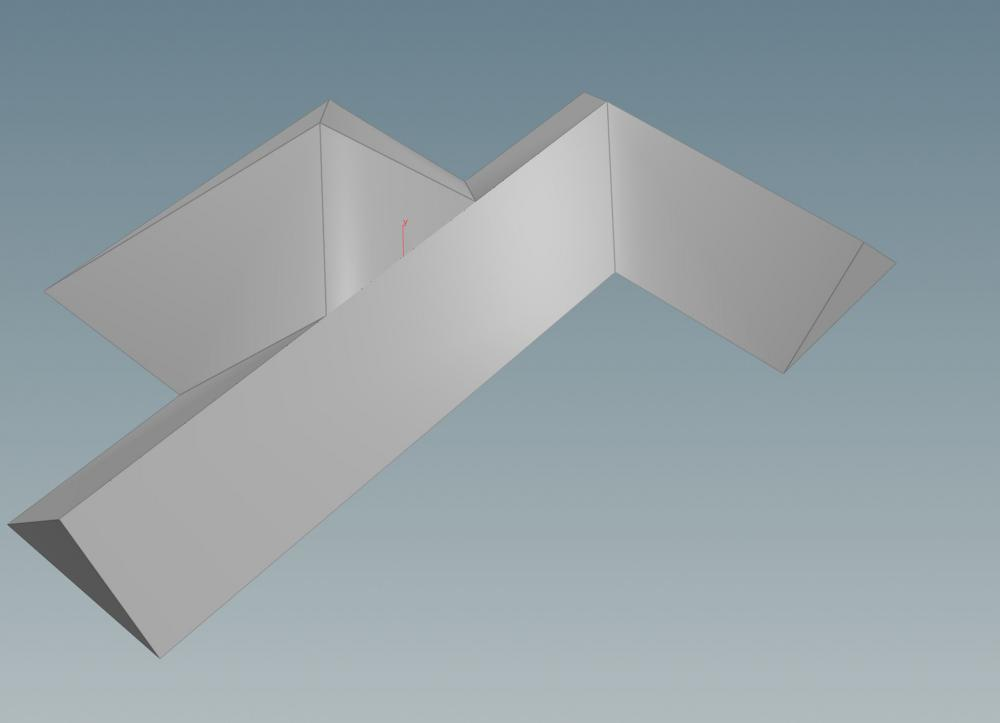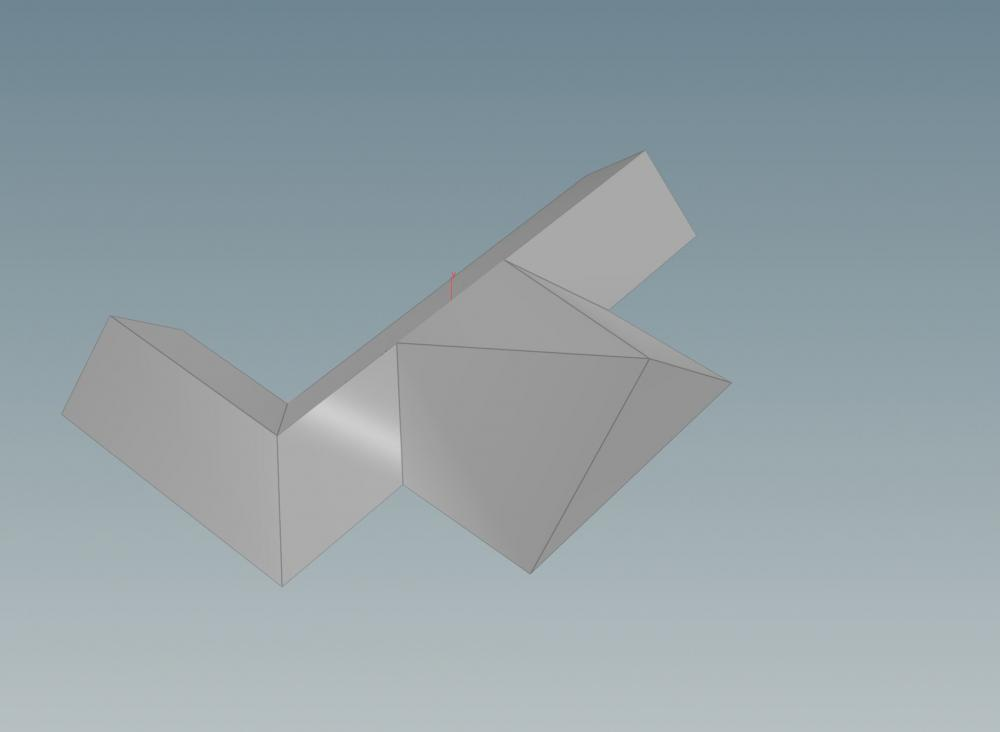RoofCornerproblem.hiplc

##### Share on other sites

Hi tmac,

you can move the internal point of a triangle to the border by applying the average position of its two other points to it: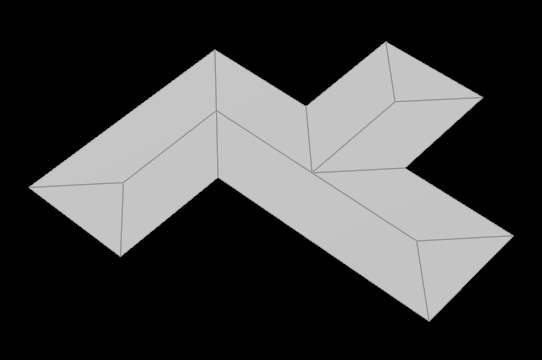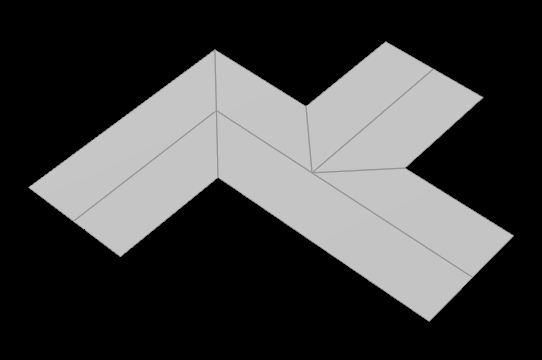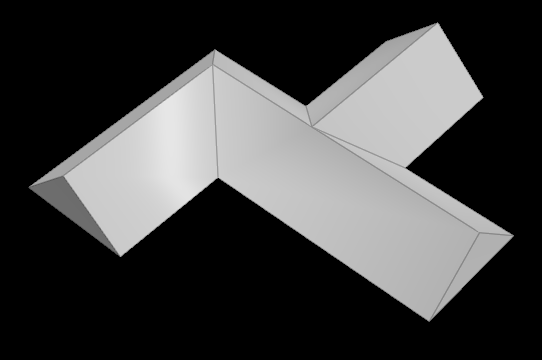```// in a primitive wrangle
if(primvertexcount(0, i@primnum) == 3){ // make sure it's a triangle
int pts[] = primpoints(0, i@primnum); // collect all points of the triangle
vector pos = 0.0; // set a initial position value for summing up the other two points positions later
int pt_move = -1; // set a initial point number for the yet unknown inner point
foreach(int pt; pts){ // run over all points of this triangle
if(inpointgroup(0, 'outer', pt) == 1){ // ask whether this point is part of the outer group
pos += point(0, 'P', pt); // add its position to the position sum from line 3
}
else{ // if the currently processed point is not part of the outer border..
pt_move = pt; // .. define it as the inner point thats supposed to be moved..
}
}
vector pos_avg = pos / 2.0; // .. to the average position of the other two points.
setpointattrib(0, 'P', pt_move, pos_avg, 'set'); // apply the new position to the inner point.
}```

Rising the roof can be done without promoting the polyframe`s egdedist attribute, simply by calling the vertex attribute within a point wrangle:

`v@P.y += vertex(0, 'edgedist', i@vtxnum);`

roof.hipnc

•2
•2

##### Share on other sites

Waw just great.  Can not thank you enough for taking the time to look at it.  You turned 17 nodes into 3.  Much appreciation.

•1

##### Share on other sites

Apologies for the inconvenience and my lack of knowledge of vex.  Just one last query regarding a point with four connecting edges that is causing an error.  Would like it deselected from the tri points as it distorts the roof shape.  I have included error scene.

roof (1).hipnc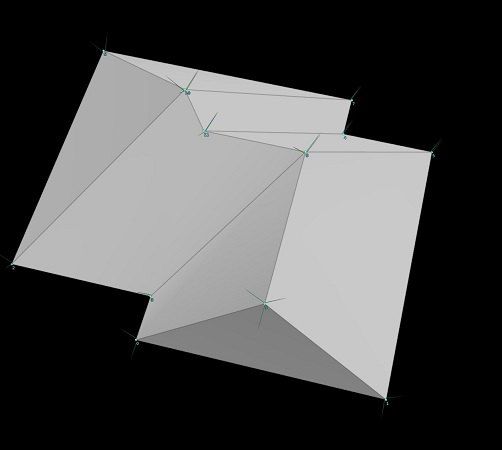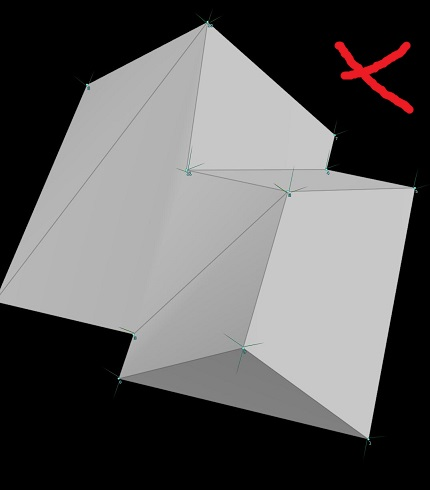##### Share on other sites

Never mind, you can check if the point has three neighbors, otherwise it does not move the point.

```if(primvertexcount(0, i@primnum) == 3){
int pts[] = primpoints(0, i@primnum);
vector pos = 0.0;
int pt_move = -1;
foreach(int pt; pts){
if(inpointgroup(0, 'outer', pt) == 1){
pos += point(0, 'P', pt);
}
else{
pt_move = pt;
}
}
if( neighbourcount(0, pt_move) == 3){
vector pos_avg = pos * chf('shape');
setpointattrib(0, 'P', pt_move, pos_avg, 'set');
}
}```

##### Share on other sites
•1

## Create an account

Register a new account